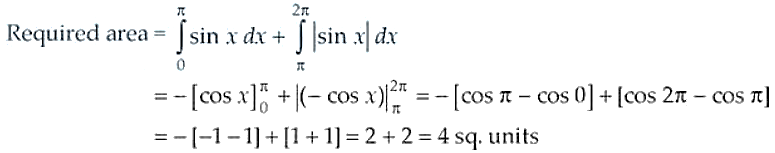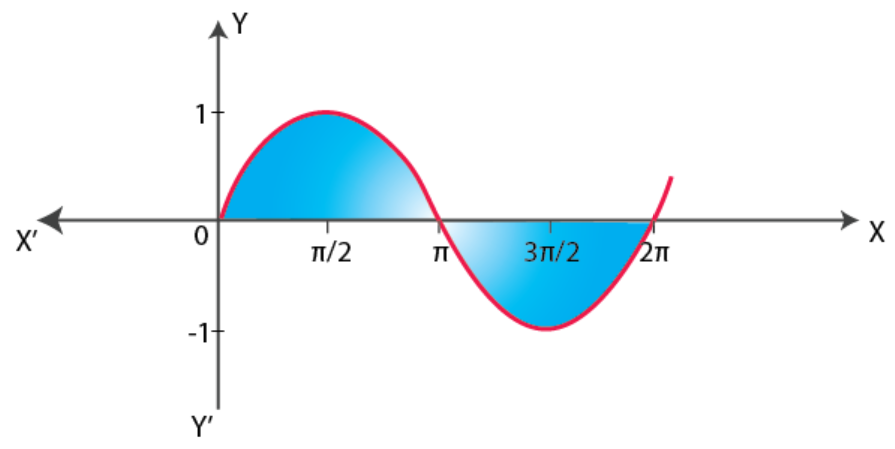# NCERT Exemplar Solutions for Class 12 Maths Chapter 8 Application of Integrals

NCERT Exemplar problems are important for students in their exam preparations. They will get advanced knowledge of the subjects, which is important from an examination point of view. The NCERT Exemplar Solutions have been developed to solve the exercise problems in the chapters. Also, these solutions are according to the latest CBSE guidelines.

The 8th chapter of NCERT Exemplar Solutions for Class 12 Maths is the Application of Integrals. In this chapter, students will learn the application of integrals with the method of finding the area under simple curves, the area of the region bounded by a curve and a line and area between two curves. Students who want to clear their doubts and understand how to solve the exercise problems in a step-by-step manner can access the solutions PDF of NCERT Exemplar Solutions for Class 12 Maths Chapter 8 Application of Integrals, available in the link below.

## Download the PDF of NCERT Exemplar Solutions for Class 12 Maths Chapter 8 Application of Integrals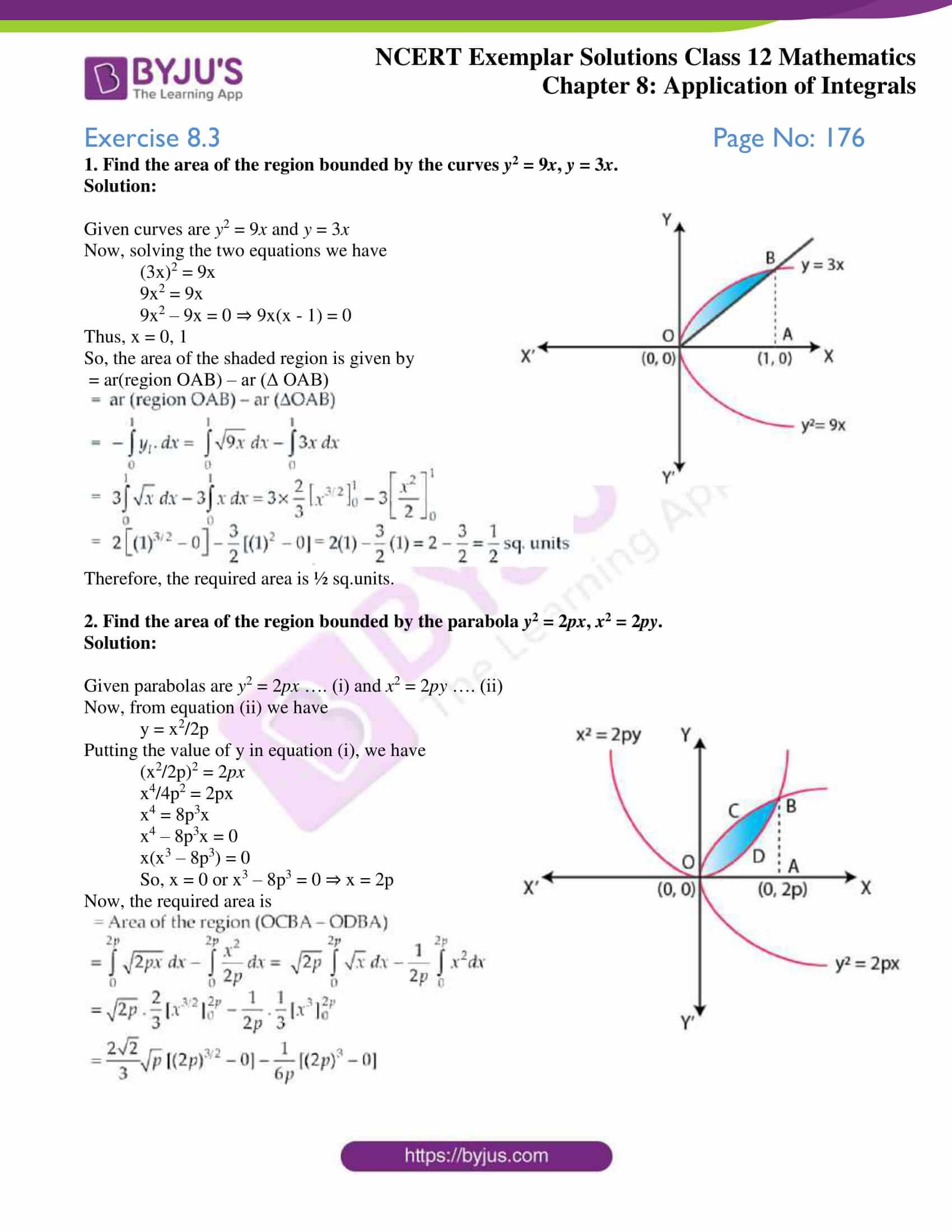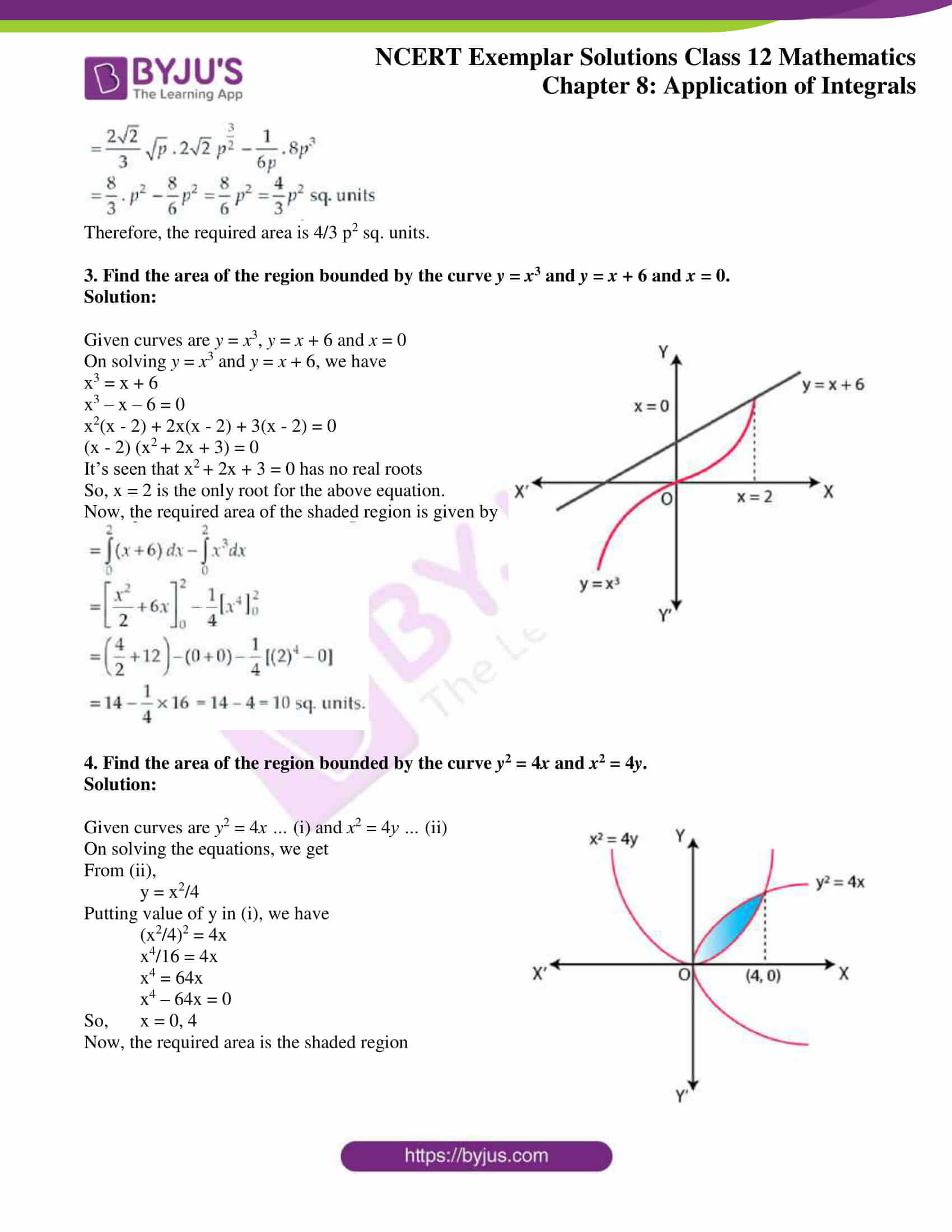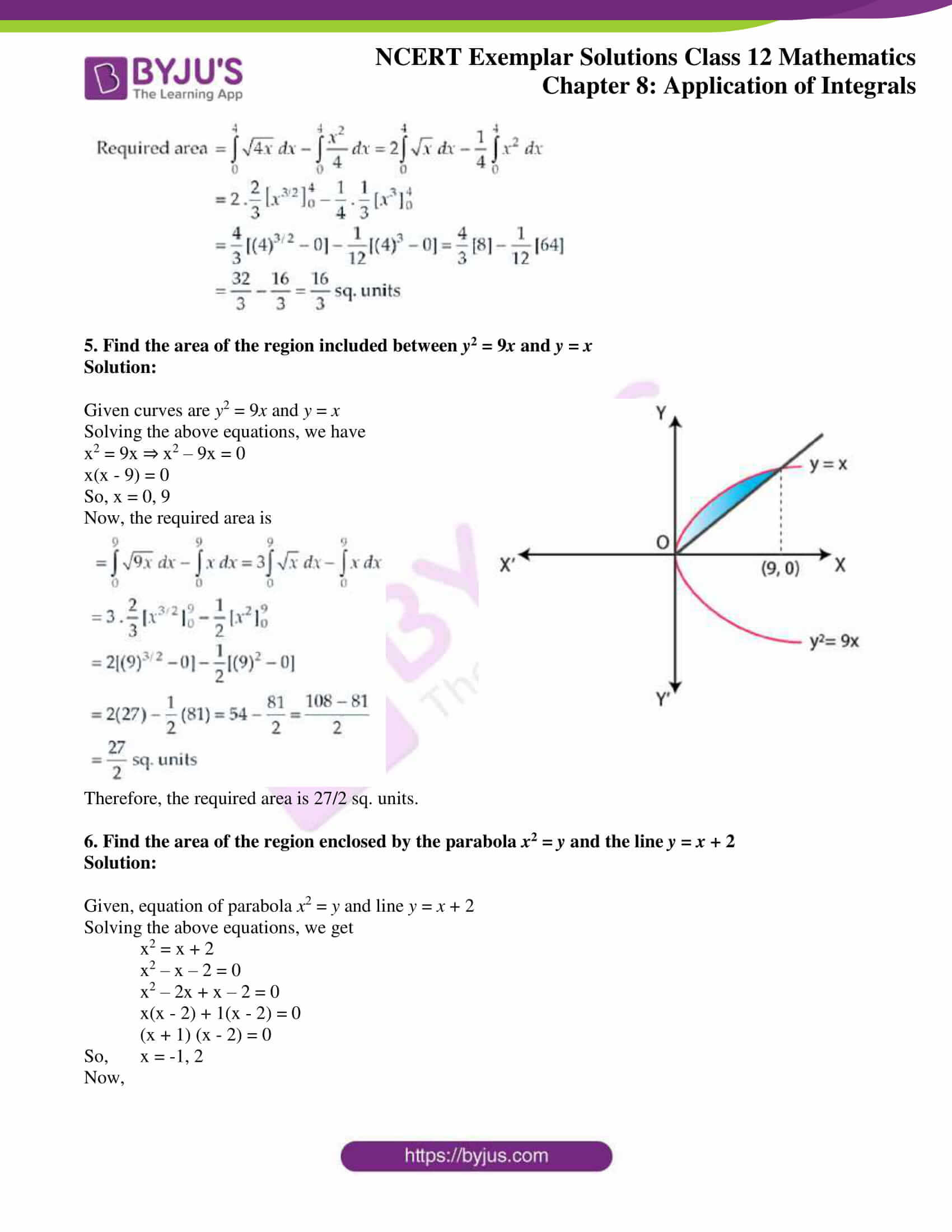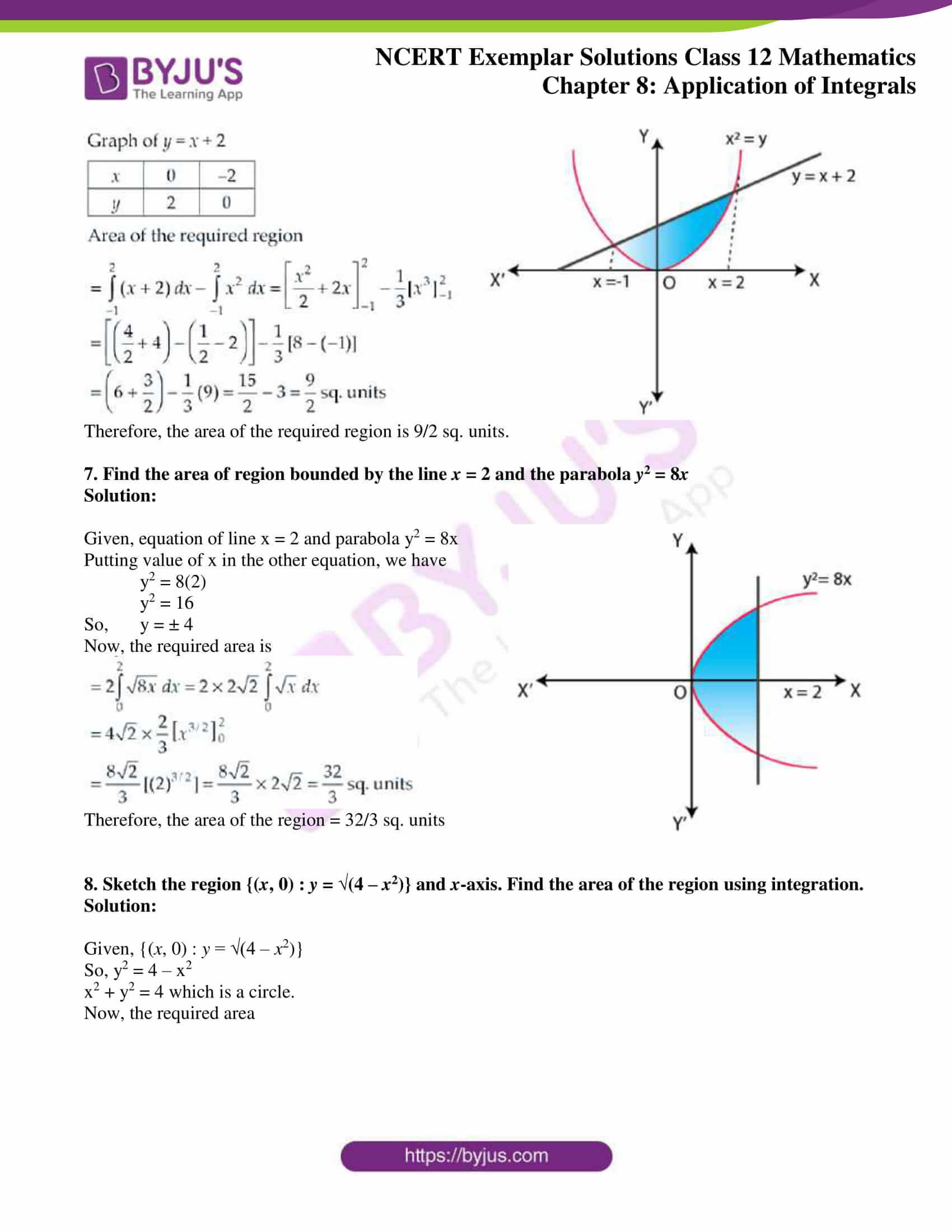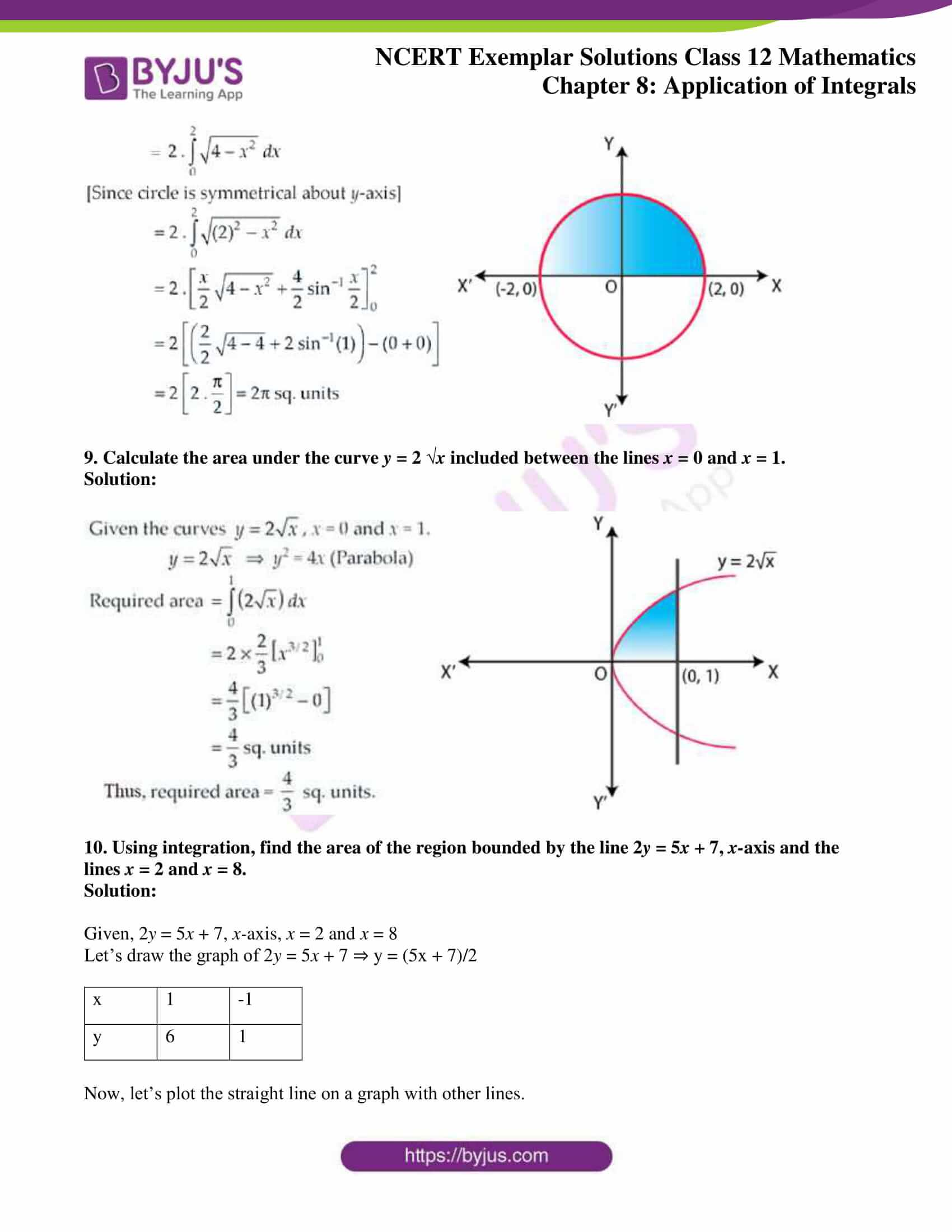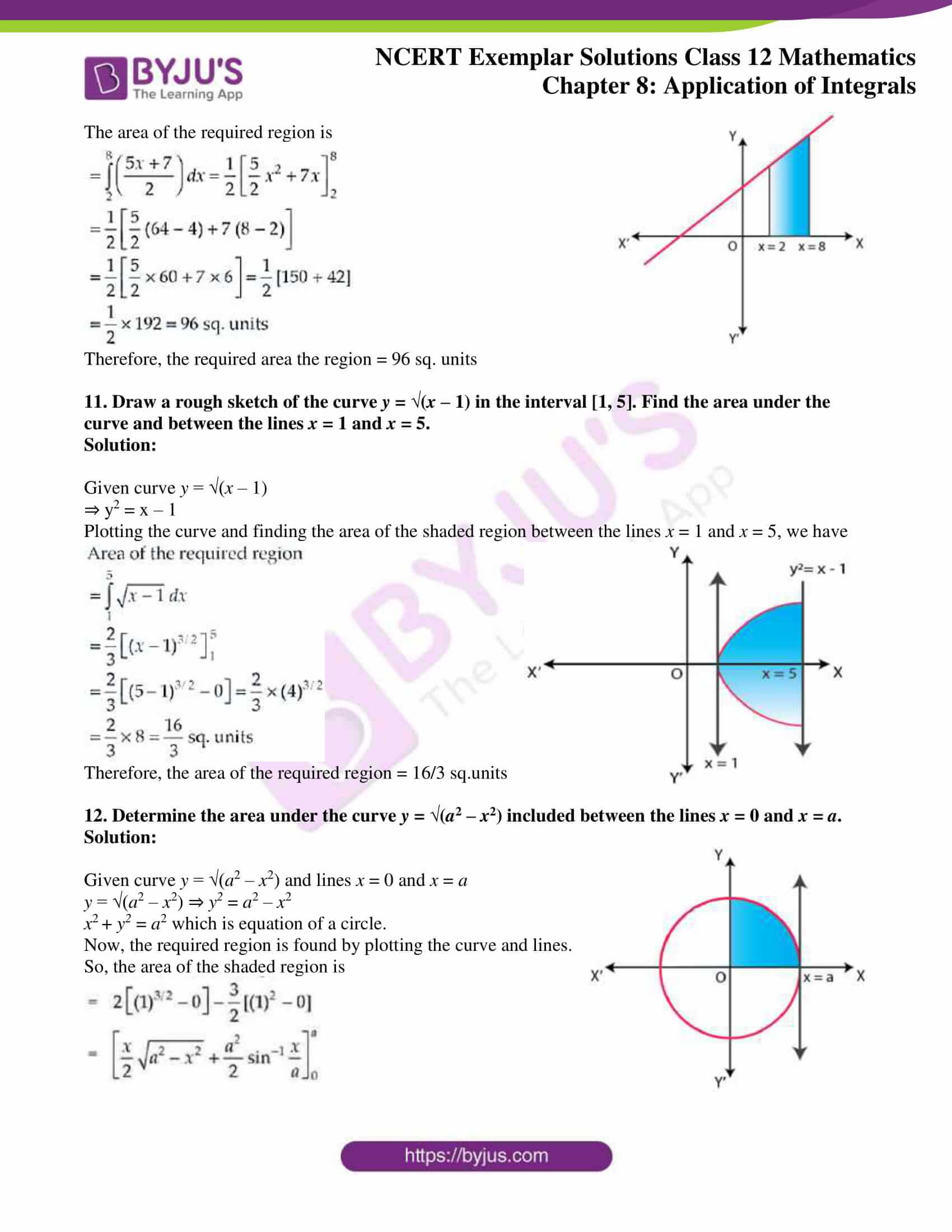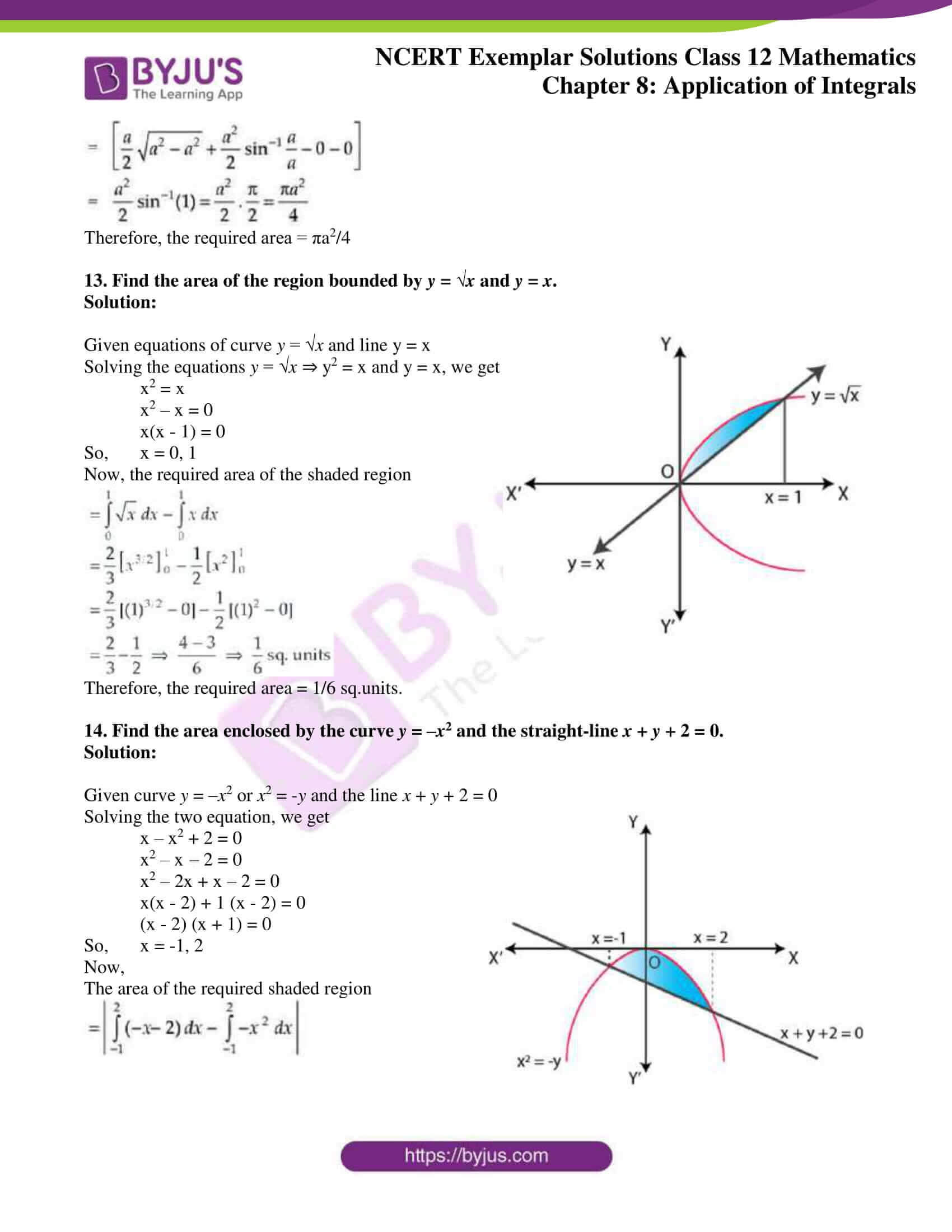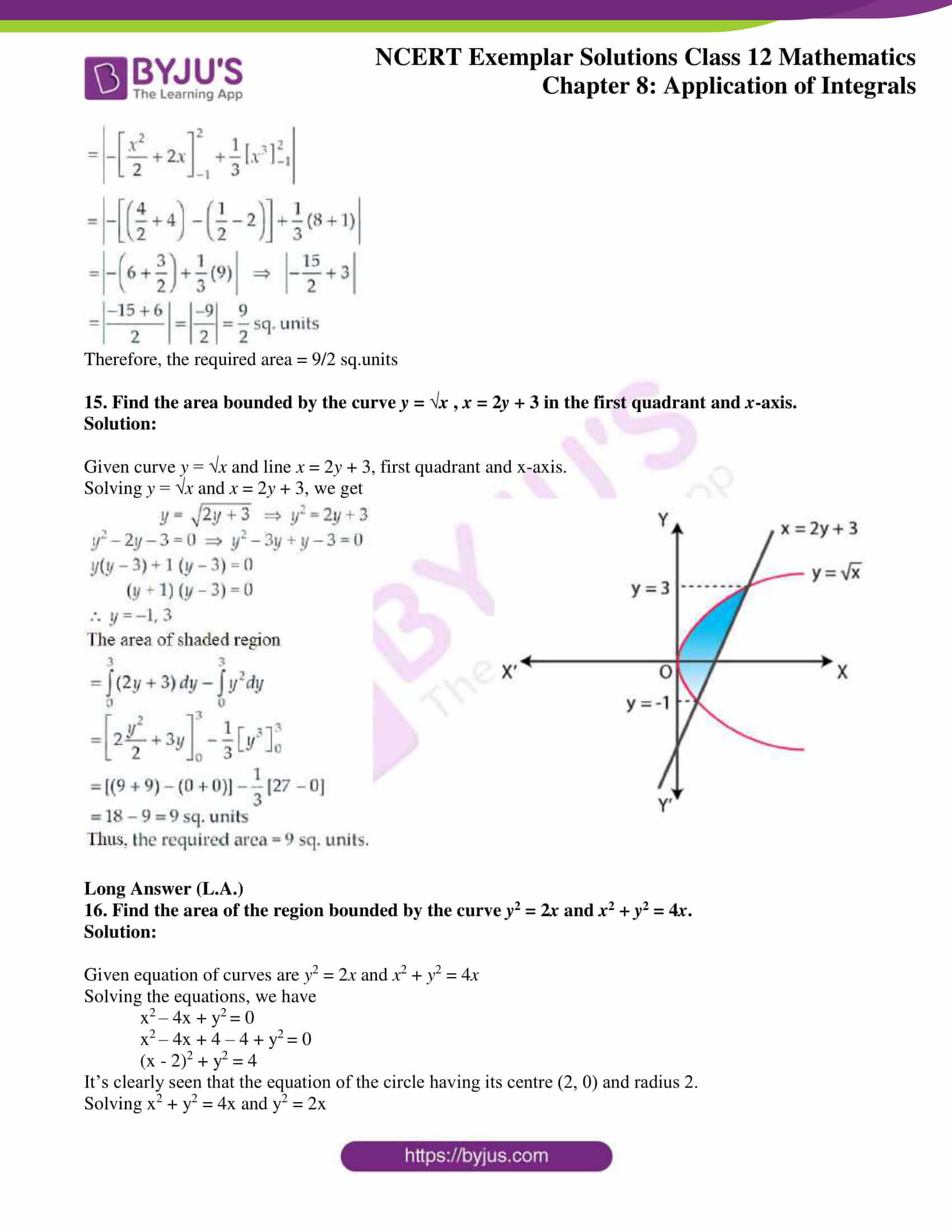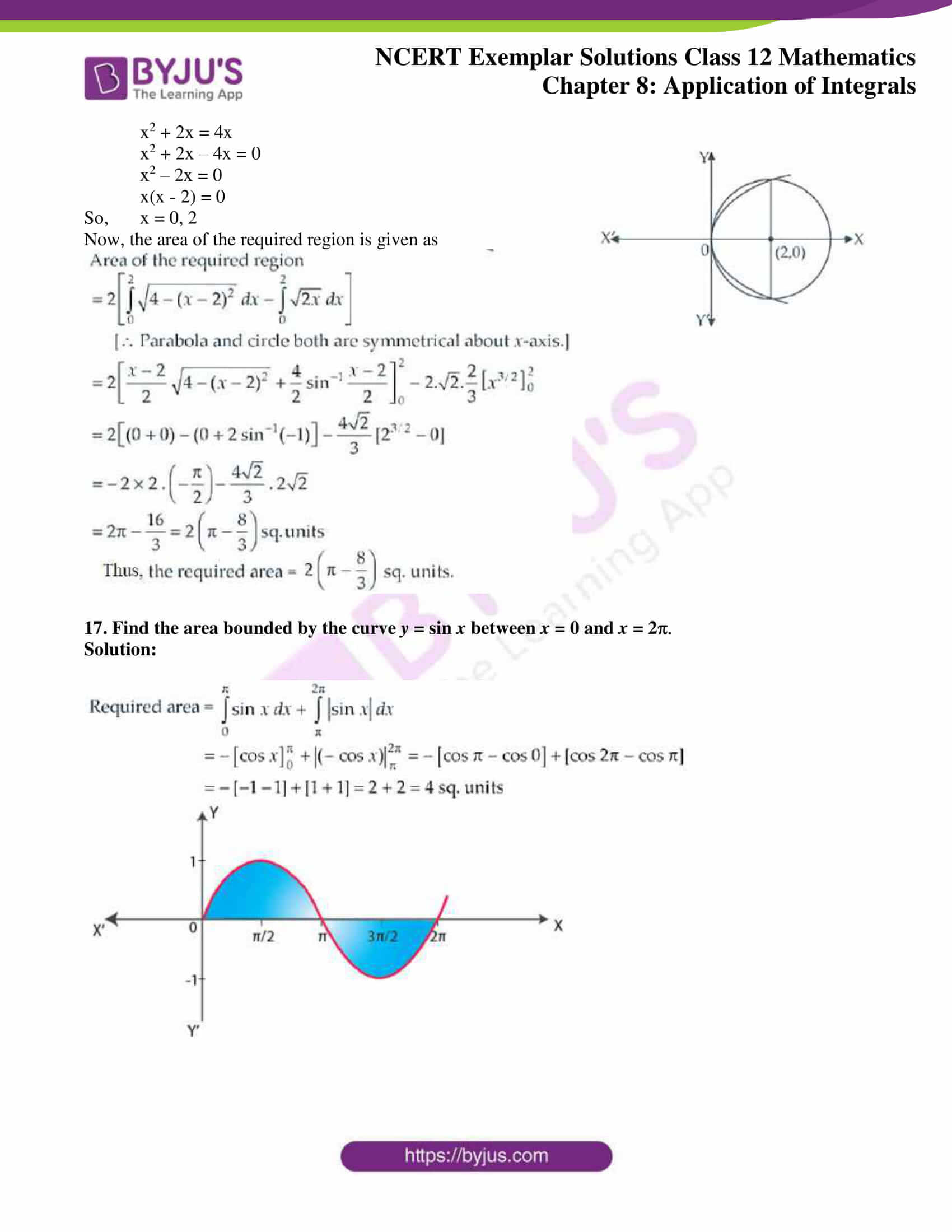### Access Answers to NCERT Exemplar Class 12 Maths Chapter 8 Application of Integrals

Exercise 8.3 Page No: 176

1. Find the area of the region bounded by the curves y2 = 9x, y = 3x.

Solution: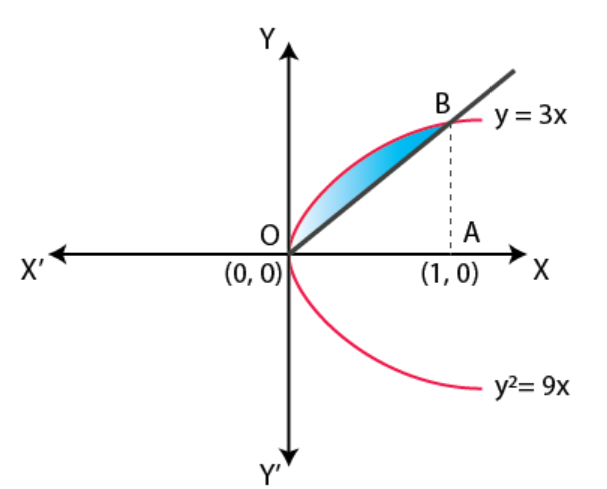Given curves are y2 = 9x and y = 3x

Now, solving the two equations we have

(3x)2 = 9x

9x2 = 9x

9x2 – 9x = 0 ⇒ 9x(x – 1) = 0

Thus, x = 0, 1

So, the area of the shaded region is given by

= ar(region OAB) – ar (Δ OAB)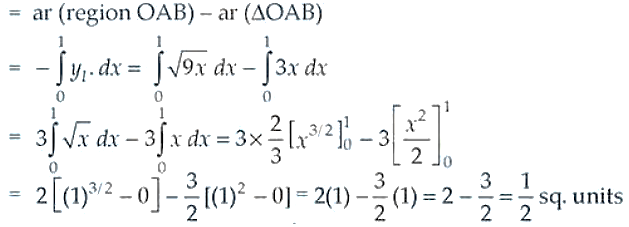Therefore, the required area is ½ sq.units.

2. Find the area of the region bounded by the parabola y2 = 2px, x2 = 2py.

Solution:

Given parabolas are y2 = 2px …. (i) and x2 = 2py …. (ii)

Now, from equation (ii) we have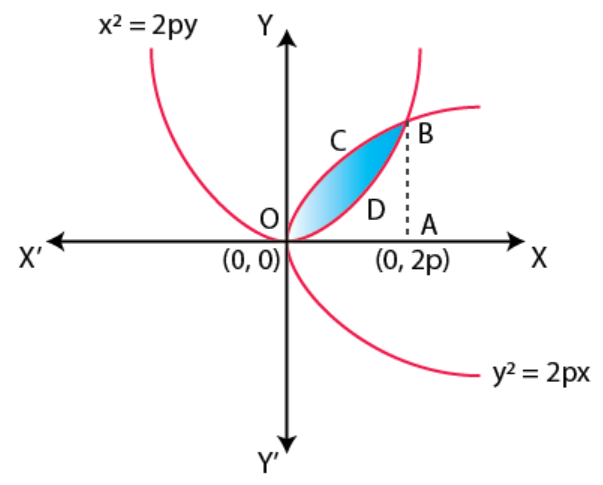y = x2/2p

Putting the value of y in equation (i), we have

(x2/2p)2 = 2px

x4/4p2 = 2px

x4 = 8p3x

x4 – 8p3x = 0

x(x3 – 8p3) = 0

So, x = 0 or x3 – 8p3 = 0 ⇒ x = 2p

Now, the required area is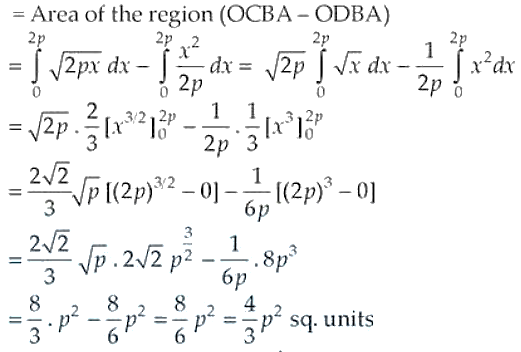Therefore, the required area is 4/3 p2 sq. units.

3. Find the area of the region bounded by the curve y = x3 and y = x + 6 and x = 0.

Solution: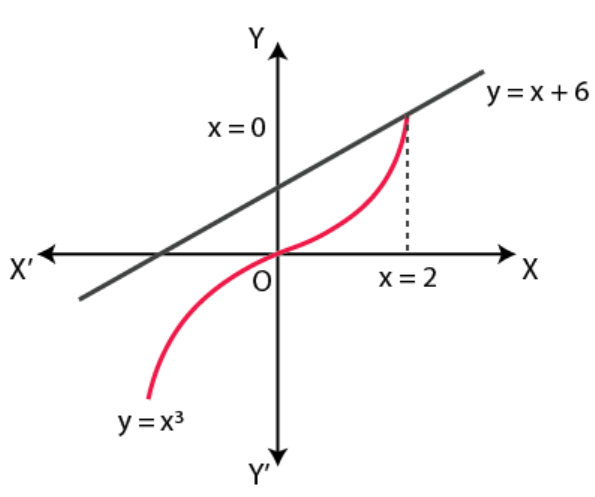Given curves are y = x3, y = x + 6 and x = 0

On solving y = x3 and y = x + 6, we have

x3 = x + 6

x3 – x – 6 = 0

x2(x – 2) + 2x(x – 2) + 3(x – 2) = 0

(x – 2) (x2 + 2x + 3) = 0

It’s seen that x2 + 2x + 3 = 0 has no real roots

So, x = 2 is the only root for the above equation.

Now, the required area of the shaded region is given by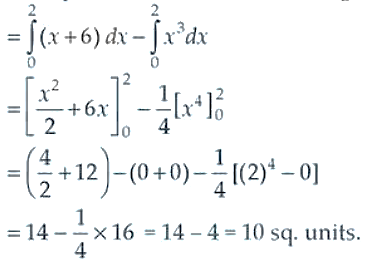4. Find the area of the region bounded by the curve y2 = 4x and x2 = 4y.

Solution: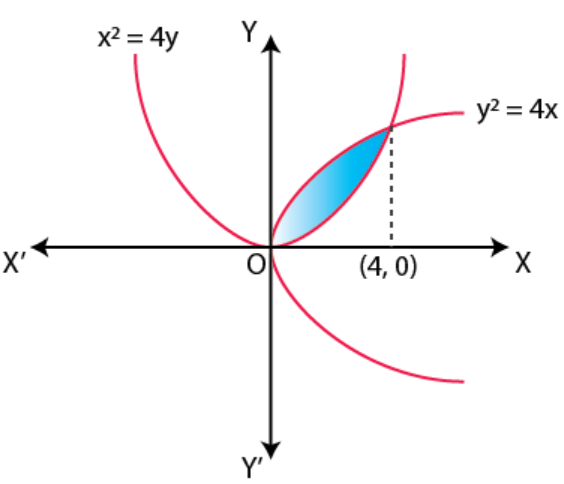Given curves are y2 = 4x … (i) and x2 = 4y … (ii)

On solving the equations, we get

From (ii),

y = x2/4

Putting value of y in (i), we have

(x2/4)2 = 4x

x4/16 = 4x

x4 = 64x

x4 – 64x = 0

So, x = 0, 4

Now, the required area is the shaded region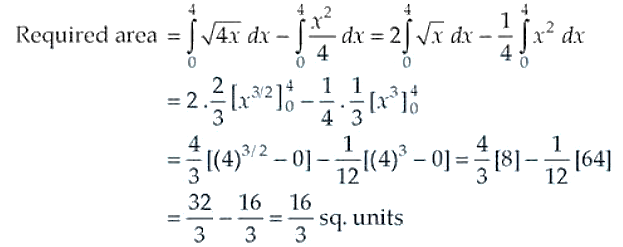5. Find the area of the region included between y2 = 9x and y = x

Solution: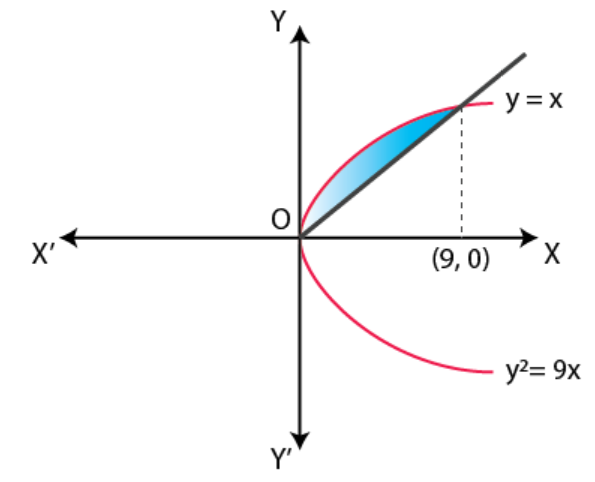Given curves are y2 = 9x and y = x

Solving the above equations, we have

x2 = 9x ⇒ x2 – 9x = 0

x(x – 9) = 0

So, x = 0, 9

Now, the required area is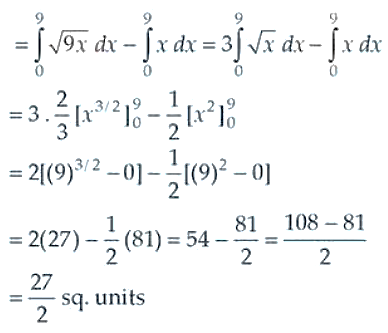Therefore, the required area is 27/2 sq. units.

6. Find the area of the region enclosed by the parabola x2 = y and the line y = x + 2

Solution:

Given, equation of parabola x2 = y and line y = x + 2

Solving the above equations, we get

x2 = x + 2

x2 – x – 2 = 0

x2 – 2x + x – 2 = 0

x(x – 2) + 1(x – 2) = 0

(x + 1) (x – 2) = 0

So, x = -1, 2

Now,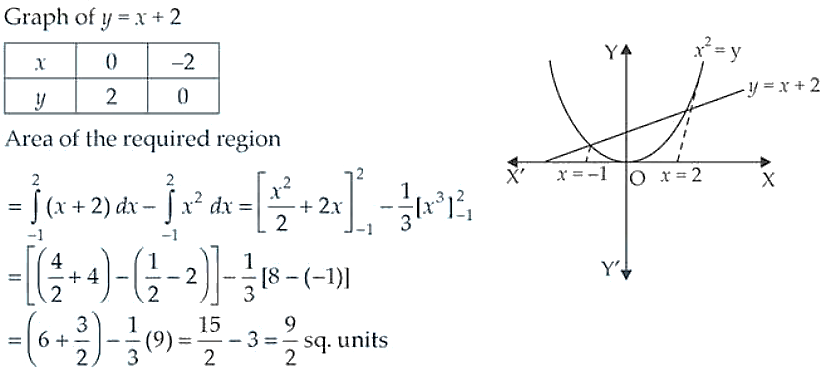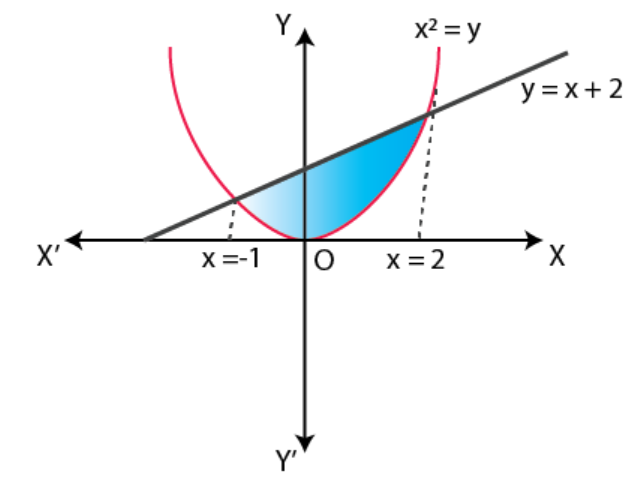Therefore, the area of the required region is 9/2 sq. units.

7. Find the area of region bounded by the line x = 2 and the parabola y2 = 8x

Solution: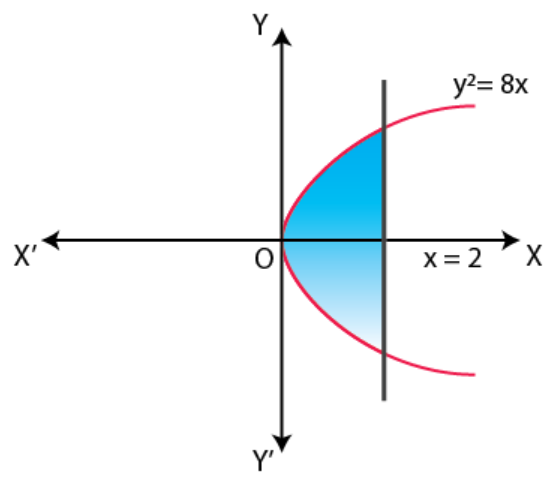Given, equation of line x = 2 and parabola y2 = 8x

Putting value of x in the other equation, we have

y2 = 8(2)

y2 = 16

So, y = ± 4

Now, the required area is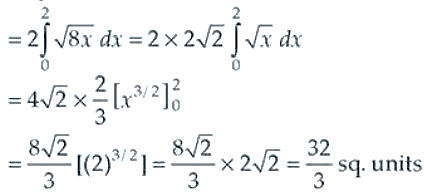Therefore, the area of the region = 32/3 sq. units

8. Sketch the region {(x, 0) : y = √(4 – x2)} and x-axis. Find the area of the region using integration.

Solution:

Given, {(x, 0) : y = √(4 – x2)}

So, y2 = 4 – x2

x2 + y2 = 4 which is a circle.

Now, the required area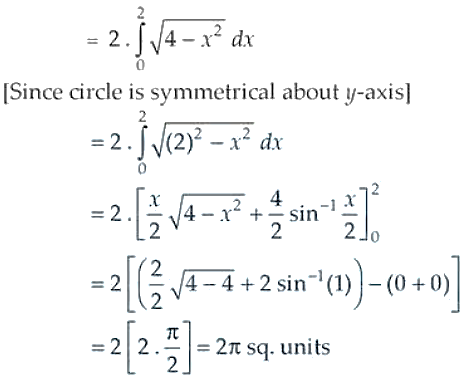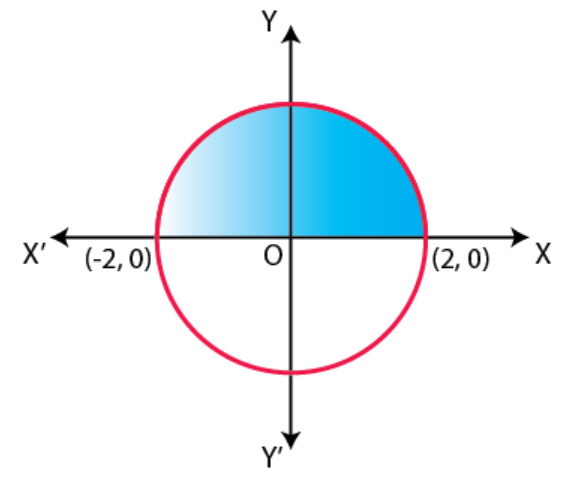9. Calculate the area under the curve y = 2 √x included between the lines x = 0 and x = 1.

Solution: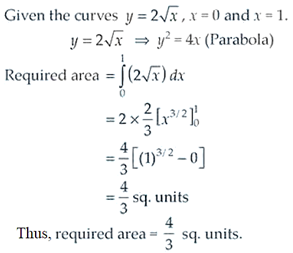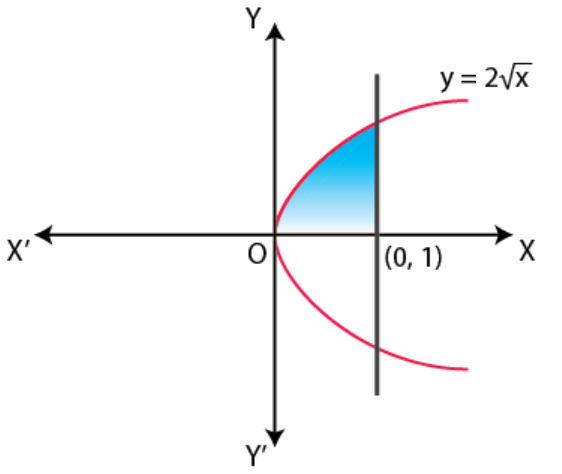10. Using integration, find the area of the region bounded by the line 2y = 5x + 7, x-axis and the lines x = 2 and x = 8.

Solution:

Given, 2y = 5x + 7, x-axis, x = 2 and x = 8

Let’s draw the graph of 2y = 5x + 7 ⇒ y = (5x + 7)/2

 x 1 -1 y 6 1

Now, let’s plot the straight line on a graph with other lines.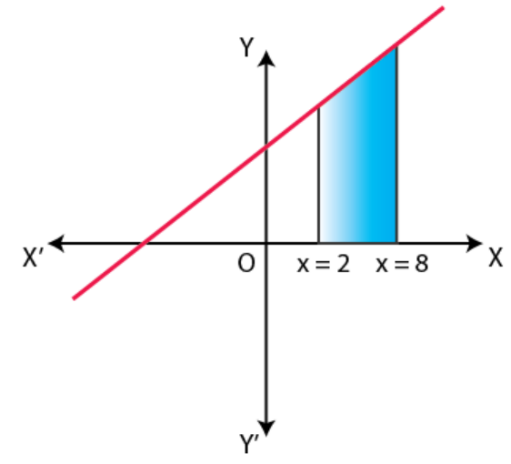The area of the required region is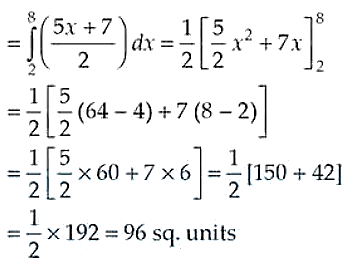Therefore, the required area the region = 96 sq. units

11. Draw a rough sketch of the curve y = √(x – 1) in the interval [1, 5]. Find the area under the curve and between the lines x = 1 and x = 5.

Solution:

Given curve y = √(x – 1)

⇒ y2 = x – 1

Plotting the curve and finding the area of the shaded region between the lines x = 1 and x = 5, we have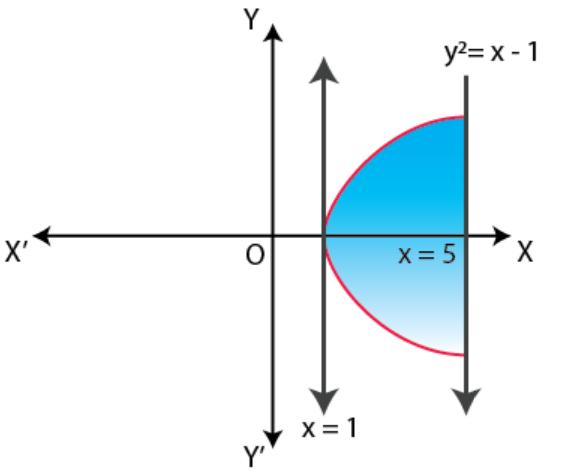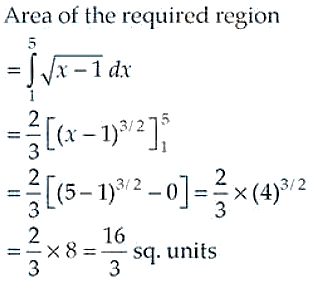Therefore, the area of the required region = 16/3 sq.units

12. Determine the area under the curve y = √(a2x2) included between the lines x = 0 and x = a.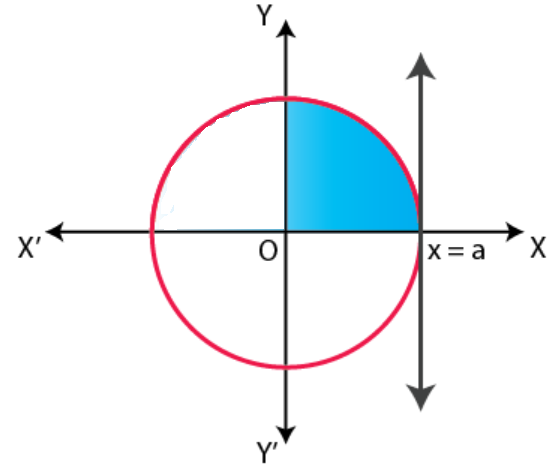Solution:

Given curve y = √(a2x2) and lines x = 0 and x = a

y = √(a2x2) ⇒ y2 = a2x2

x2 + y2 = a2 which is equation of a circle.

Now, the required region is found by plotting the curve and lines.

So, the area of the shaded region is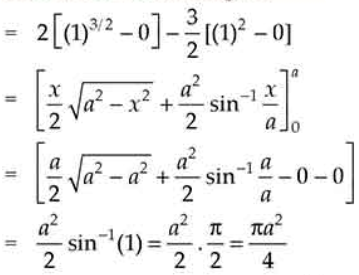Therefore, the required area = πa2/4

13. Find the area of the region bounded by y = √x and y = x.

Solution: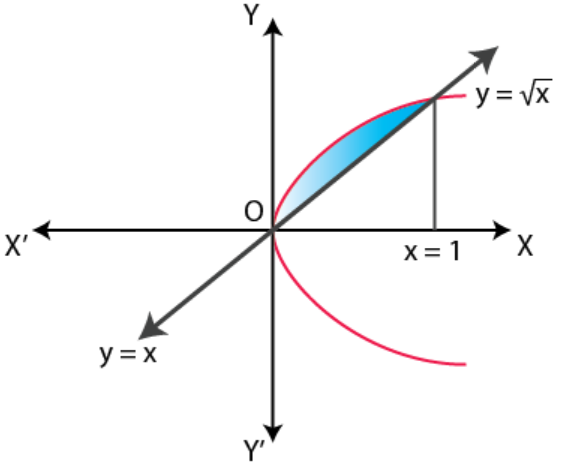Given equations of curve y = √x and line y = x

Solving the equations y = √x ⇒ y2 = x and y = x, we get

x2 = x

x2 – x = 0

x(x – 1) = 0

So, x = 0, 1

Now, the required area of the shaded region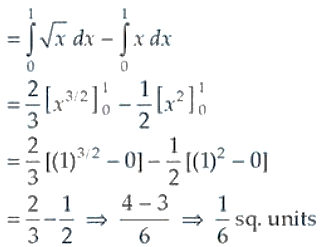Therefore, the required area = 1/6 sq.units.

14. Find the area enclosed by the curve y = –x2 and the straight-line x + y + 2 = 0.

Solution:

Given curve y = –x2 or x2 = –y and the line x + y + 2 = 0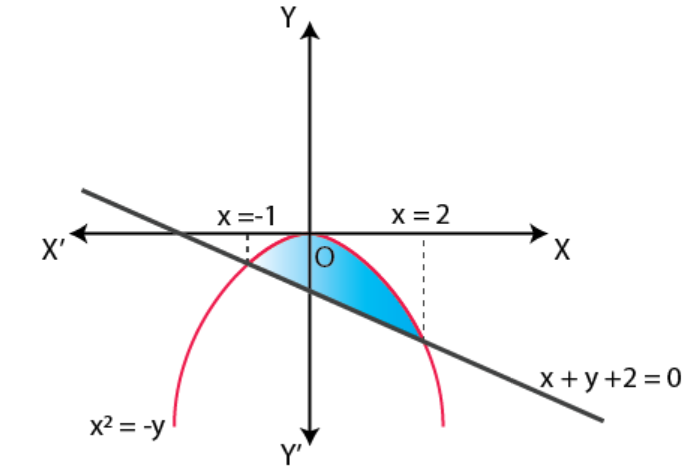Solving the two equation, we get

x – x2 + 2 = 0

x2 – x – 2 = 0

x2 – 2x + x – 2 = 0

x(x – 2) + 1 (x – 2) = 0

(x – 2) (x + 1) = 0

So, x = -1, 2

Now,

The area of the required shaded region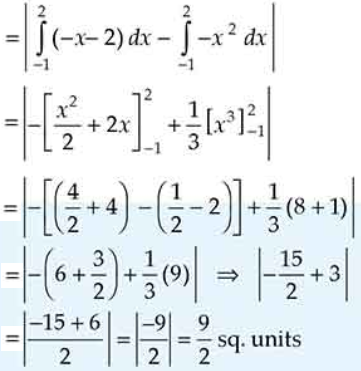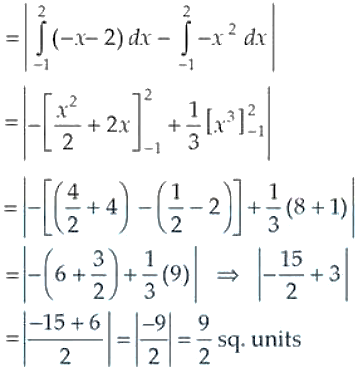Therefore, the required area = 9/2 sq.units

15. Find the area bounded by the curve y = √x , x = 2y + 3 in the first quadrant and x-axis.

Solution:

Given curve y = √x and line x = 2y + 3, first quadrant and x-axis.

Solving y = √x and x = 2y + 3, we get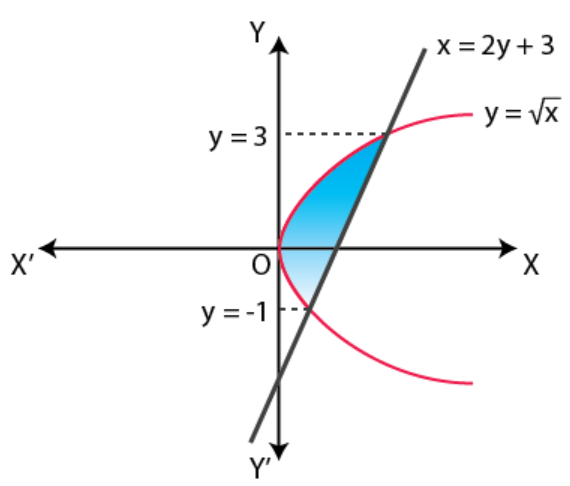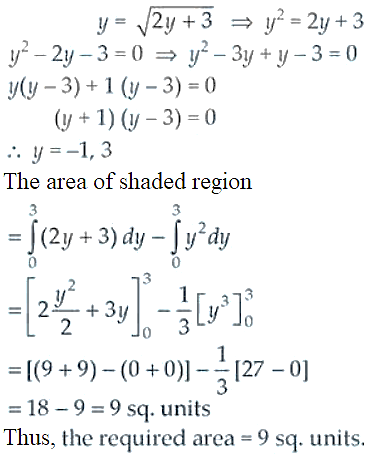16. Find the area of the region bounded by the curve y2 = 2x and x2 + y2 = 4x.

Solution:

Given equation of curves are y2 = 2x and x2 + y2 = 4x

Solving the equations, we have

x2 – 4x + y2 = 0

x2 – 4x + 4 – 4 + y2 = 0

(x – 2)2 + y2 = 4

It’s clearly seen that the equation of the circle having its centre (2, 0) and radius 2.

Solving x2 + y2 = 4x and y2 = 2x

x2 + 2x = 4x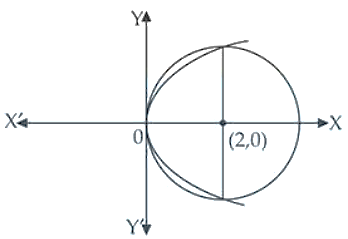x2 + 2x – 4x = 0

x2 – 2x = 0

x(x – 2) = 0

So, x = 0, 2

Now, the area of the required region is given as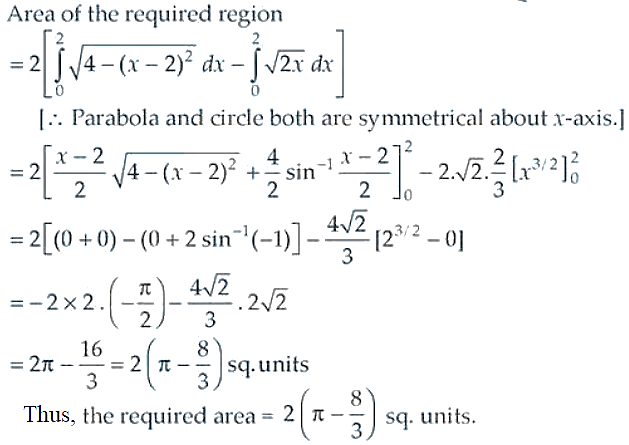17. Find the area bounded by the curve y = sin x between x = 0 and x = 2p.

Solution: